### 5.5Toward High Performance STT-MRAM-Based Stateful Logic

The energy consumptions and the average error probabilities of the same Boolean logic functions are calculated by using the improved MTJs SPICE model and the reliability analysis method explained in Chapter 4. The same device (MTJ and transistor) characteristics are assumed for both implementations and the circuit parameters are optimized for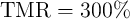with respect to their minimum error probabilities of the basic logic operations (Fig. 4.21).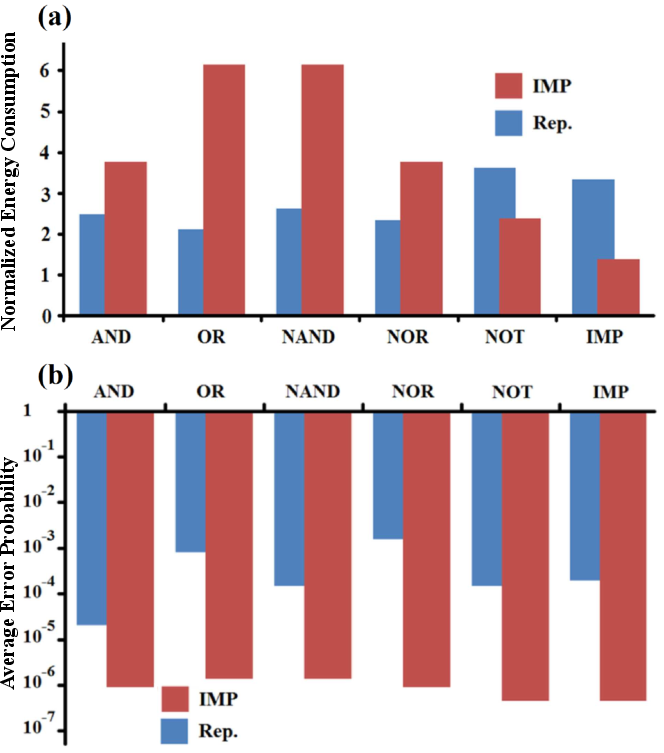Figure 5.8.: (a) Normalized energy consumption and (b) minimum average error probabilities plotted for MRAM-based implication (IMP) and reprogrammable (Rep.) implementations of some basic Boolean logic operations. The energy is normalized by the TRUE operation switching energy which is equal to 18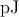for a pulse duration of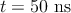in the simulations.

Fig. 5.8a shows the energy consumptions of implication- and reprogrammable-based implementations of some basic Boolean logic operations. The energy consumptions are normalized by the amount of energy required for AP–to–P MTJ switching which is equal to 18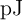for pulse duration of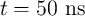in the simulations. Due to the mismatch between the intrinsic logic functions of the gates, the implication-based implementation requires more steps and thus more energy (by an average factor of1.4) to implement the basic operations. However, even for the intrinsic functions of the reprogrammable gate, the implication logic exhibits about 1-3 orders of magnitude higher reliability than the reprogrammable logic architecture (Fig. 5.8b).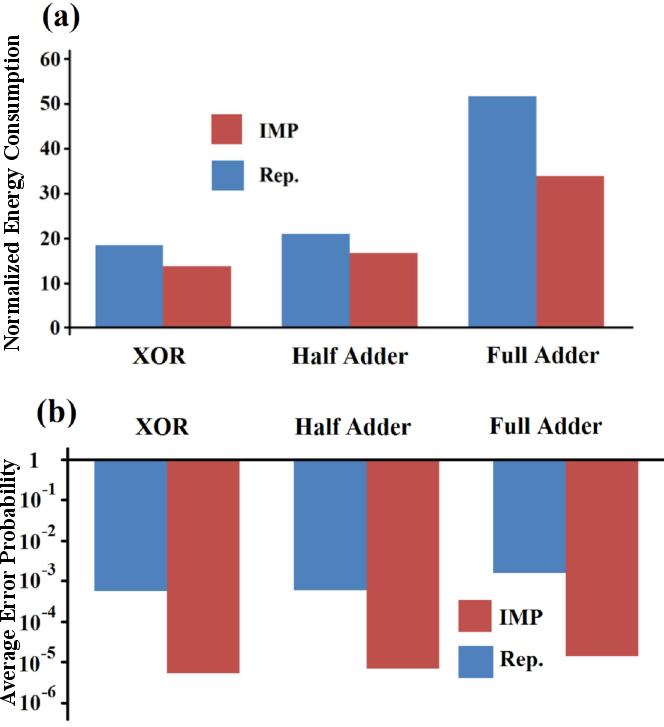Figure 5.9.: (a) Normalized energy consumption and (b) minimum average error probabilities plotted for different logic functions.

In order to see the performance at larger circuits, the energy consumptions and the average error probabilities of more complex Boolean functions including an XOR, a half adder, and a full adder are compared in Fig. 5.9a and Fig. 5.9b. Only AND and NAND operations are used to provide the most reliable design for the reprogrammable architecture. Nevertheless, Fig. 5.9b shows that the implication-based implementation of more complex functions exhibits about two orders of magnitude higher reliability than the most reliable design with the reprogrammable architecture. Furthermore, for complex logic functions which are not inherently covered by the gates, the implication logic architecture performs also better with respect to the power consumption (Fig. 5.9a). In combination with ‘0’ and ‘1’ writing operations, both reprogrammable-based AND-NAND and implication-based IMP-NIMP logic functions form complete logic bases. Thus, any Boolean logic function can be computed in a series of subsequent steps using these architectures. Furthermore, combining implication and (N)AND-based reprogrammable frameworks in MRAM arrays is a possible direction in designing large-scalable MRAM-based logic circuits featuring a minimized number of logic steps and an optimized error, delay, and power consumption.

#### 5.5.1Combined Reprogrammable-Implication Logic

The implication logic outperforms the reprogrammable architecture in most aspects as explained before (Fig. 5.9) and thus is expected to be the implementation of choice for MRAM-based stateful logic circuits. However, in the following more design tradeoffs are discussed by combining these logic architectures.

Due to their computational completeness, implication and reprogrammable MRAM-based stateful logic architectures can be used independently to design any Boolean logic function. However, their combination can minimize the number of required logic steps as it provides more degrees of freedom by employing more fundamental logic operations and choosing the gates with the best performance with respect to their desired attributes. Thus, the execution time and the energy consumption of complex logic functions can be reduced. For example, in the combined reprogrammable-implication (CRI) architecture the XOR function can be designed as:

 TRUE : a3,a4 = 1 NIMP : a3 → a1 ≡{a3′ = a 3.a1 = a1} NIMP : a4 → a2 ≡{a4′ = a 4.a2 = a2} Preset : b1 = 0 NAND : b1 = a1.a4 Preset : b2 = 0 NAND : b2 = a2.a3 Preset : a5 = 1 AND : a5 = b1.b2 ≡ a1 XOR a2, (5.14)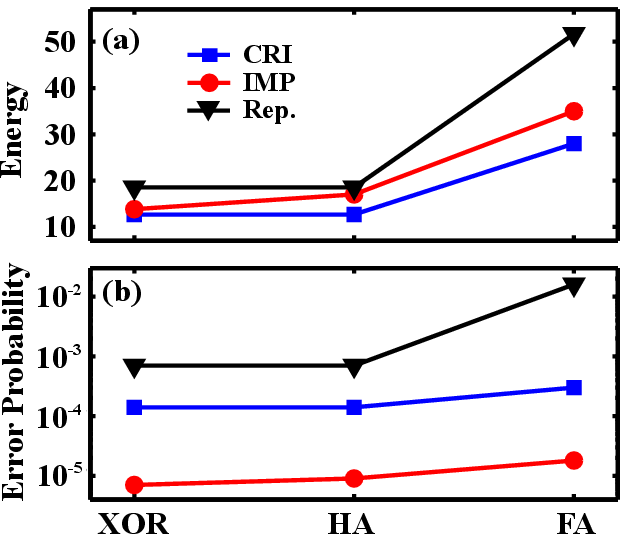Figure 5.10.: (a) Energy consumption for complex logic functions. (b)for different MRAM-based implementations of functions XOR, half adder (HA), and full adder (FA).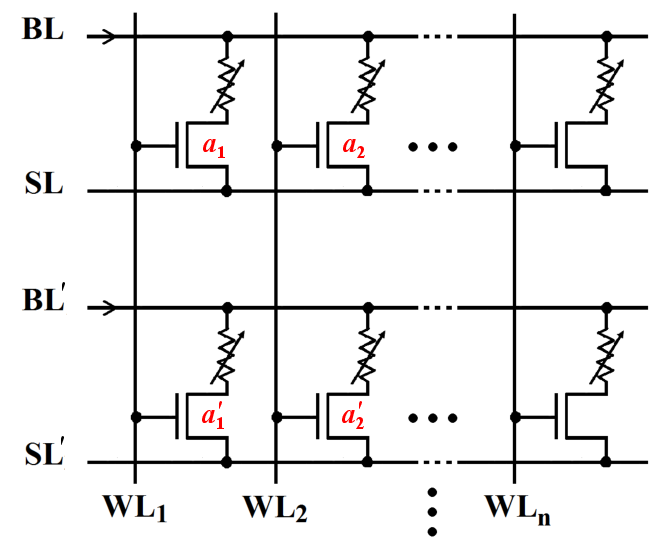Figure 5.11.: Coupled MRAM arrays based on the common STT-MRAM architecture suited for parallel MRAM-based computations.

According to Fig. 5.10a, the CRI design provides a lower energy consumption compared to both implication and reprogrammable designs. However, its error probabilities is higher than the implication design (Fig. 5.10b) since it employs basic reprogrammable operations.

#### 5.5.2Parallel STT-MRAM-Based Computation

Parallelization of several MRAM arrays can be used to simultaneously perform operations on the same word lines to decrease the number of required serial steps. For example, the required steps to implement the XOR function presented in Eq. 5.7 can be performed in parallel in the MRAM structure shown in Fig. 5.11. By applying the single/dual mode voltage signals to the word lines (Fig. 5.6b) to execute TRUE/NIMP (FALSE/IMP) operations, the corresponding MTJs are selected or pre-selected in all arrays. Therefore, by applying relevant current signals (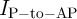or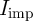) simultaneously to all current-carrying lines (SLs and BLs), the computations are performed in parallel. This significantly reduces the total time needed for implementing XOR functions on binary data (and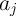) stored in the-th and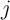-th MTJs of each array. Similarly, reprogrammable operations can be parallelized when the two group of coupled arrays are connected in series.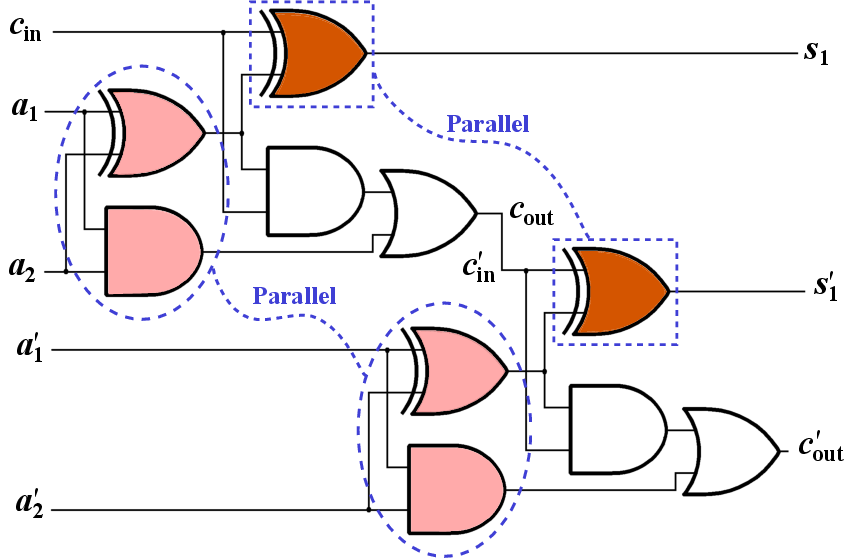Figure 5.12.: Logic diagram of a two-bit full adder.

For more complicated applications in which intermediate results have to be used as input for the next logic steps (e.g.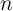-bit full adders, where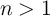) only some parts of the computations can be performed in parallel. As an example, Fig. 5.12 shows a two-bit full adder in which the carry output from the first full adder (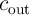) is connected to the carry input of the second full adder (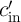). Therefore, it is not possible to perform all computations in parallel.

Assuming that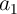(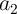) and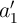(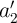) are stored in the first (second) MTJs in the MRAM arrays 1 and 1which have coupled WLs, the AND and the XOR functions between (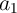,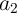) and (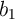,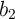) can be performed in parallel (Fig. 5.12). In fact, when for performing a logic operation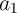andare selected or pre-selected through the corresponding WLs,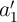andare also selected or pre-selected as they share the same WL with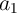and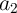, respectively. Therefore, if the same current pulses are applied to both BL and BL, the same logic operations will be performed in parallel in both arrays. After performing the first AND and XOR operations in parallel,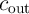(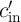) is calculated without parallelization. This part of the calculations is performed in Array 1 and it cannot be parallelized with other computations in Array 1. Then by using a read/write operation the result is written into the MTJ in Array 1which is in the same WL as the MTJ that holds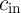in Array 1. After that, the last XOR functions are performed in parallel to calculate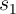and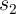and finally the calculations are continued to compute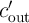in Array 1. As a result, by parallelization of the MRAM-based logic arrays, the total calculation time required for the implication-based and CRI-based implementations of a two-bit full adder are decreased by about 40% and 50% (Fig. 5.13). It is worth noting that the parallelization does not affect the reliability and the energy consumption since only some steps are performed in parallel while the total number of logic operations is still fixed.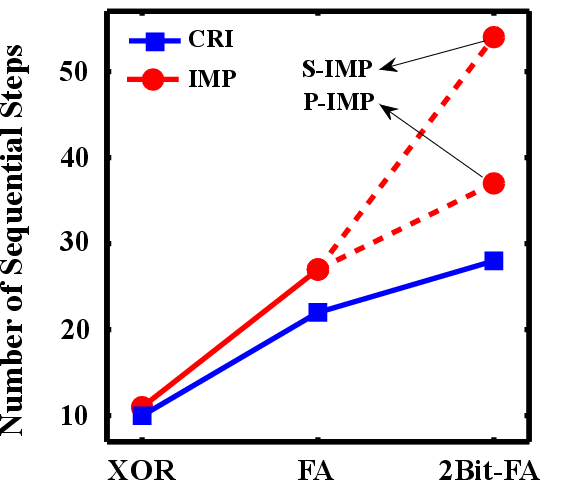Figure 5.13.: Required sequential steps for serial (S-IMP) and parallel (P-IMP) MRAM-based implication and combined reprogrammable-implication (CRI) architectures.